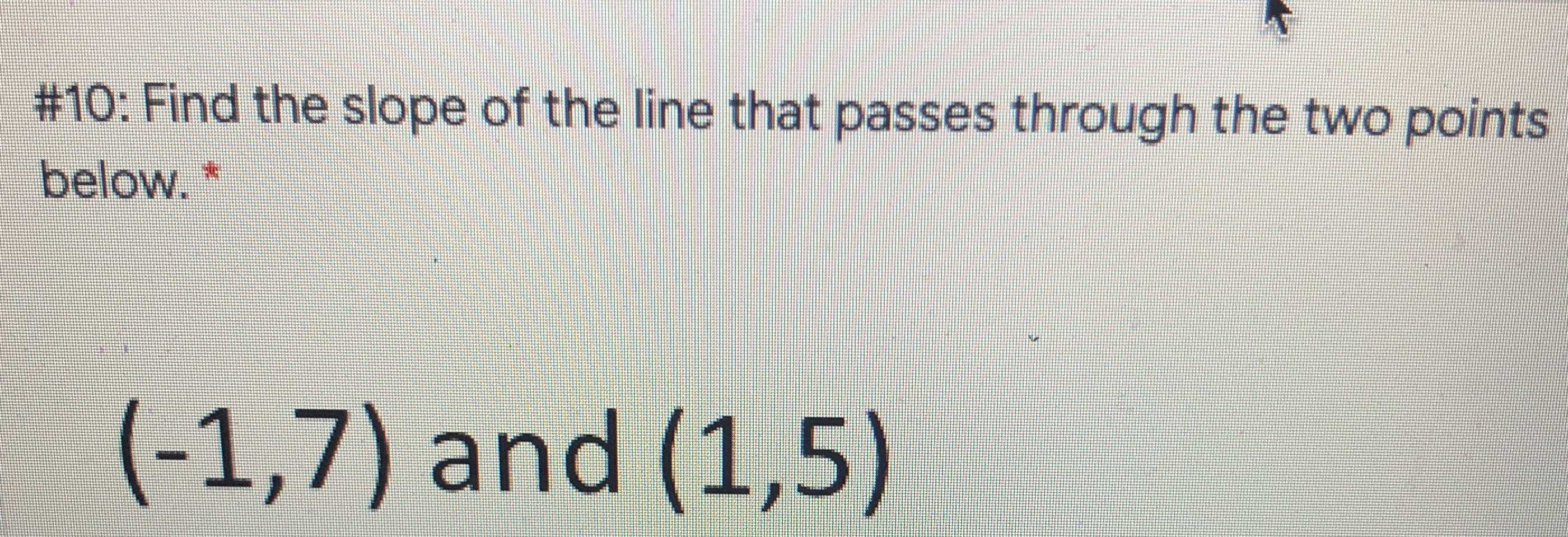### ¿Todavía tienes preguntas de matemáticas?

Pregunte a nuestros tutores expertos
Algebra
Pregunta#10: Find the slope of the line that passes through the two points below. $$^ { * }$$

$$( - 1,7 )$$ a\bigcap $$( 1,5 )$$